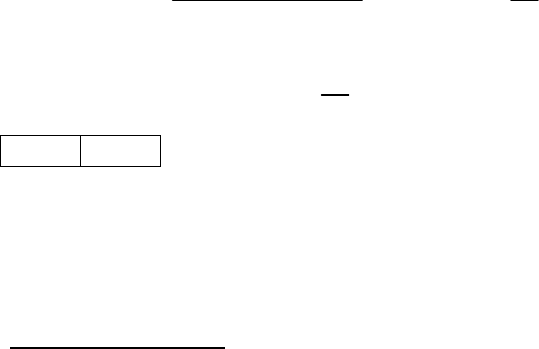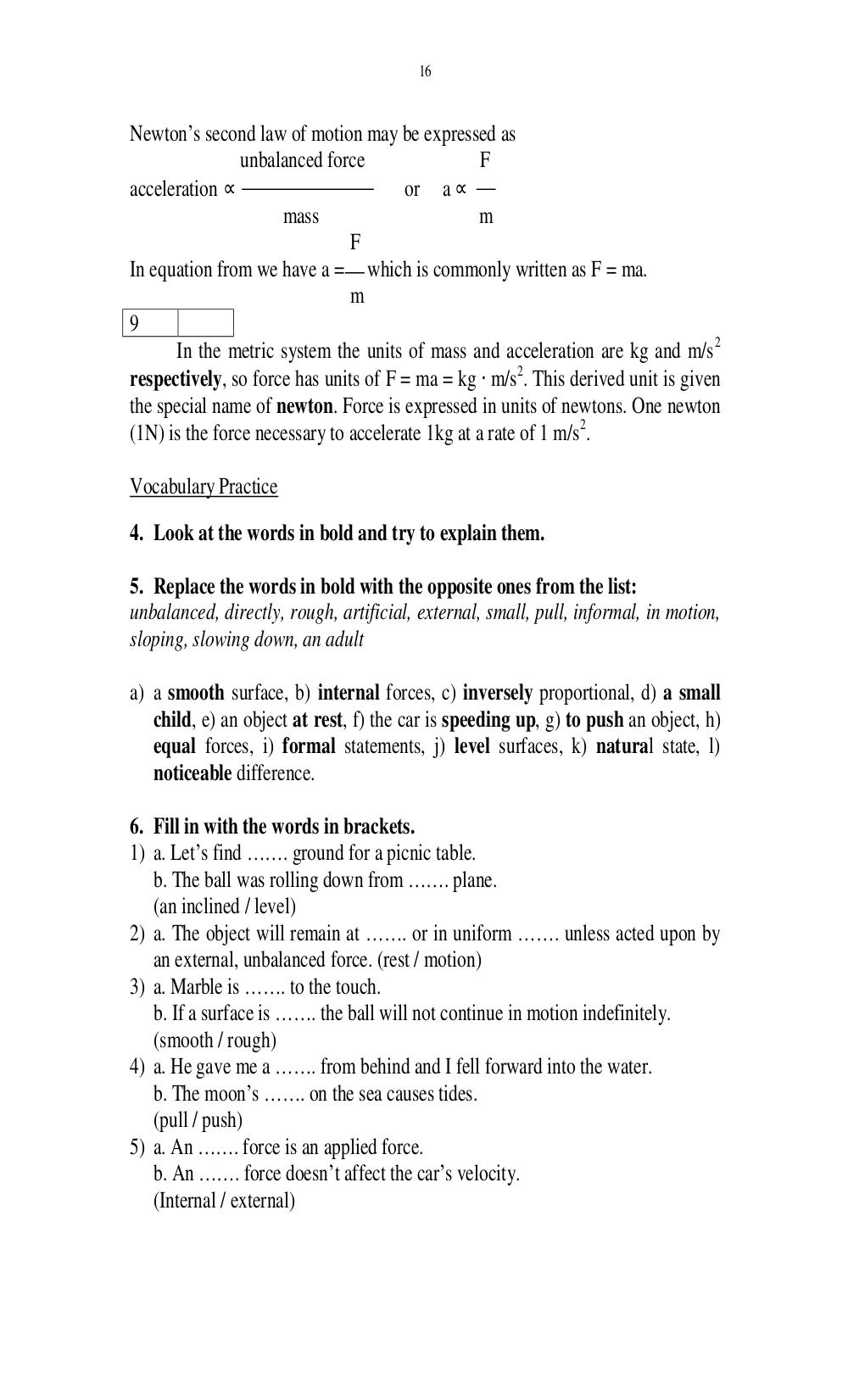# Английский язык. Ильичева Н.А - 16 стр.

Составители:

Рубрика:

• ## Иностранный язык16
Newtons second law of motion may be expressed as
unbalanced force F
acceleration or a
mass m
F
In equation from we have a = which is commonly written as F = ma.
m
9
In the metric system the units of mass and acceleration are kg and m/s
2
respectively, so force has units of F = ma = kg m/s
2
. This derived unit is given
the special name of newton. Force is expressed in units of newtons. One newton
(1N) is the force necessary to accelerate 1kg at a rate of 1 m/s
2
.
Vocabulary Practice
4. Look at the words in bold and try to explain them.
5. Replace the words in bold with the opposite ones from the list:
unbalanced, directly, rough, artificial, external, small, pull, informal, in motion,
a) a smooth surface, b) internal forces, c) inversely proportional, d) a small
child, e) an object at rest, f) the car is speeding up, g) to push an object, h)
equal forces, i) formal statements, j) level surfaces, k) natural state, l)
noticeable difference.
6. Fill in with the words in brackets.
1) a. Lets find ……. ground for a picnic table.
b. The ball was rolling down from ……. plane.
(an inclined / level)
2) a. The object will remain at ……. or in uniform ……. unless acted upon by
an external, unbalanced force. (rest / motion)
3) a. Marble is ……. to the touch.
b. If a surface is ……. the ball will not continue in motion indefinitely.
(smooth / rough)
4) a. He gave me a ……. from behind and I fell forward into the water.
b. The moons ……. on the sea causes tides.
(pull / push)
5) a. An ……. force is an applied force.
b. An ……. force doesnt affect the cars velocity.
(Internal / external)16

Newton’s second law of motion may be expressed as
unbalanced force                  F
acceleration ∝                         or a ∝
mass                        m
F
In equation from we have a = which is commonly written as F = ma.
m
9
In the metric system the units of mass and acceleration are kg and m/s 2
respectively, so force has units of F = ma = kg ⋅ m/s2. This derived unit is given
the special name of newton. Force is expressed in units of newtons. One newton
(1N) is the force necessary to accelerate 1kg at a rate of 1 m/s 2.

Vocabulary Practice
4. Look at the words in bold and try to explain them.

5. Replace the words in bold with the opposite ones from the list:
unbalanced, directly, rough, artificial, external, small, pull, informal, in motion,

a) a smooth surface, b) internal forces, c) inversely proportional, d) a small
child, e) an object at rest, f) the car is speeding up, g) to push an object, h)
equal forces, i) formal statements, j) level surfaces, k) natural state, l)
noticeable difference.

6. Fill in with the words in brackets.
1) a. Let’s find ……. ground for a picnic table.
b. The ball was rolling down from ……. plane.
(an inclined / level)
2) a. The object will remain at ……. or in uniform ……. unless acted upon by
an external, unbalanced force. (rest / motion)
3) a. Marble is ……. to the touch.
b. If a surface is ……. the ball will not continue in motion indefinitely.
(smooth / rough)
4) a. He gave me a ……. from behind and I fell forward into the water.
b. The moon’s ……. on the sea causes tides.
(pull / push)
5) a. An ……. force is an applied force.
b. An ……. force doesn’t affect the car’s velocity.
(Internal / external)